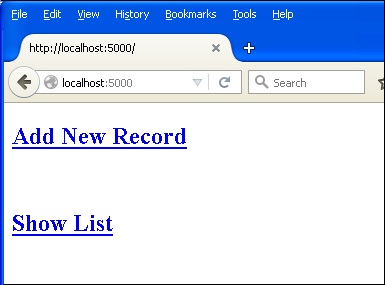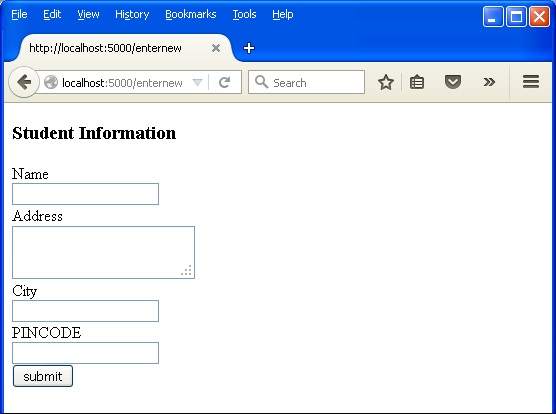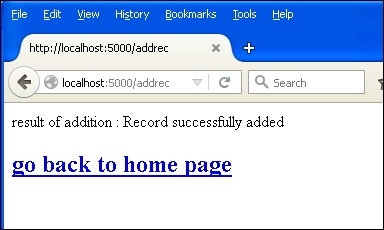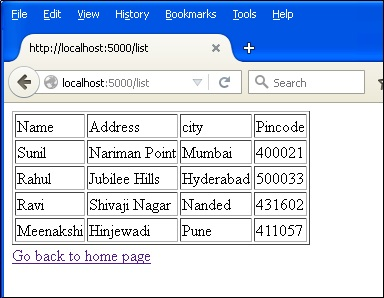Python has an in-built support for SQlite. SQlite3 module is shipped with Python distribution. For a detailed tutorial on using SQLite database in Python, please refer to this link. In this section we shall see how a Flask application interacts with SQLite.

Create an SQLite database ŌĆśdatabase.dbŌĆÖ and create a studentsŌĆÖ table in it.

```import sqlite3

conn = sqlite3.connect('database.db')
print "Opened database successfully";

conn.execute('CREATE TABLE students (name TEXT, addr TEXT, city TEXT, pin TEXT)')
print "Table created successfully";
conn.close()
```

Our Flask application has three View functions.

First new_student() function is bound to the URL rule (ŌĆś/addnewŌĆÖ). It renders an HTML file containing student information form.

```@app.route('/enternew')
def new_student():
return render_template('student.html')
```

The HTML script for ŌĆśstudent.htmlŌĆÖ is as follows −

```<html>
<body>
<form action = "{{ url_for('addrec') }}" method = "POST">
<h3>Student Information</h3>
Name<br>
<input type = "text" name = "nm" /></br>

City<br>
<input type = "text" name = "city" /><br>

PINCODE<br>
<input type = "text" name = "pin" /><br>
<input type = "submit" value = "submit" /><br>
</form>
</body>
</html>
```

As it can be seen, form data is posted to the ŌĆś/addrecŌĆÖ URL which binds the addrec() function.

This addrec() function retrieves the formŌĆÖs data by POST method and inserts in students table. Message corresponding to success or error in insert operation is rendered to ŌĆśresult.htmlŌĆÖ.

```@app.route('/addrec',methods = ['POST', 'GET'])
if request.method == 'POST':
try:
nm = request.form['nm']
city = request.form['city']
pin = request.form['pin']

with sql.connect("database.db") as con:
cur = con.cursor()

con.commit()
except:
con.rollback()
msg = "error in insert operation"

finally:
return render_template("result.html",msg = msg)
con.close()
```

The HTML script of result.html contains an escaping statement {{msg}} that displays the result of Insert operation.

```<!doctype html>
<html>
<body>
result of addition : {{ msg }}
</body>
</html>
```

The application contains another list() function represented by ŌĆś/listŌĆÖ URL. It populates ŌĆśrowsŌĆÖ as a MultiDict object containing all records in the students table. This object is passed to the list.html template.

```@app.route('/list')
def list():
con = sql.connect("database.db")
con.row_factory = sql.Row

cur = con.cursor()
cur.execute("select * from students")

rows = cur.fetchall();
return render_template("list.html",rows = rows)
```

This list.html is a template, which iterates over the row set and renders the data in an HTML table.

```<!doctype html>
<html>
<body>
<table border = 1>
<td>Name</td>
<td>city</td>
<td>Pincode</td>

{% for row in rows %}
<tr>
<td>{{row["name"]}}</td>
<td> {{ row["city"]}}</td>
<td>{{row['pin']}}</td>
</tr>
{% endfor %}
</table>

</body>
</html>
```

Finally, the ŌĆś/ŌĆÖ URL rule renders a ŌĆśhome.htmlŌĆÖ which acts as the entry point of the application.

```@app.route('/')
def home():
return render_template('home.html')
```

Here is the complete code of Flask-SQLite application.

```from flask import Flask, render_template, request
import sqlite3 as sql

@app.route('/')
def home():
return render_template('home.html')

@app.route('/enternew')
def new_student():
return render_template('student.html')

if request.method == 'POST':
try:
nm = request.form['nm']
city = request.form['city']
pin = request.form['pin']

with sql.connect("database.db") as con:
cur = con.cursor()

con.commit()
except:
con.rollback()
msg = "error in insert operation"

finally:
return render_template("result.html",msg = msg)
con.close()

@app.route('/list')
def list():
con = sql.connect("database.db")
con.row_factory = sql.Row

cur = con.cursor()
cur.execute("select * from students")

rows = cur.fetchall();
return render_template("list.html",rows = rows)

if __name__ == '__main__':
app.run(debug = True)
```

Run this script from Python shell and as the development server starts running. Visit http://localhost:5000/ in browser which displays a simple menu like this −